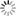# Episode List

OR

### Season 1

1961
The SailorAfter a sailor on a freighter in the port of London hits another's dog, he's viciously beaten. Bollinger has the crew restricted to their ship. Soon, the victim dies in hospital, and the killer and his dog escape into the city. They must be located quickly-it's found the dog has rabies.

1961
The HusbandBe the first one to add a plot.

1961
BessieBe the first one to add a plot.

1961
The FaceBe the first one to add a plot.

1961
The MineBe the first one to add a plot.

20 Aug. 1961
Master KeyBe the first one to add a plot.

1961
The HuntBe the first one to add a plot.

1961
The HolidayBe the first one to add a plot.

1961
The GunBe the first one to add a plot.

1961
The Jungle and Nicky SteenBe the first one to add a plot.

1961Be the first one to add a plot.

1961
The Silent WitnessBe the first one to add a plot.

21 Oct. 1961
The FrameAfter leaving prison, an ex-policeman is determined to make a witness from his trial tell the truth about the false testimony he gave, but the perjurer is murdered after an incriminating public encounter.

1961
The Othello MurderBe the first one to add a plot.

1961
The ReturnBe the first one to add a plot.

1961
The Lost KeyBe the first one to add a plot.

1961
The PoacherBe the first one to add a plot.

1961
The ScientistBe the first one to add a plot.

1961
Tomorrow's GhostAt a civil defence site, a woman is killed by a collapsed wall. Bollinger investigates, finding she was also mysteriously radioactive, and a uranium sample is suddenly two ounces lighter than it should be.

1961
Supreme CourtBe the first one to add a plot.

1961
BreakoutBe the first one to add a plot.

1961
The CountessBe the first one to add a plot.

30 Dec. 1961
SuspectBe the first one to add a plot.

1961
The ColonelBe the first one to add a plot.

1961
The EscapeBe the first one to add a plot.

1961
Inside JobBe the first one to add a plot.

1961
ConfessionBe the first one to add a plot.

1961
The WebAn older man, crippled in a wheel chair, has a young, beautiful wife, who has a secret lover. They conspire to steal her jewels and collect the insurance, but their plans don't stay secret long when Bollinger begins his inquiries.

1961
The TrackBe the first one to add a plot.

Apr. 1962
Model CrimeBe the first one to add a plot.

1961
PersuasionBe the first one to add a plot.

1961
The George Webber StoryBe the first one to add a plot.

13 Jan. 1962
The ConventionBe the first one to add a plot.

20 Jan. 1962
The AmateurBe the first one to add a plot.

1961
The AccidentBe the first one to add a plot.

1961
RunawayBe the first one to add a plot.

1961
The ContractBe the first one to add a plot.

1961
The Double AlibiBe the first one to add a plot.

3 Feb. 1962
The PaintingsBe the first one to add a plot.

Season 1  Unknown Season  »